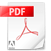## Brownian motion, stochastic calculus and Ito's formula demystified...Brownian motion, stochastic calculus and Ito's formula demystified...
In this article, you will explore the fundamental concepts of stochastic processes, Brownian motion, and Itô's formula. We will delve into the mathematical foundations of these concepts and their applications in quantitative finance and stochastic calculus. By the end of this article, you will have a basic grasp of how these powerful tools are used to model and analyze complex systems affected by randomness and uncertainty.
Brownian motion, stochastic calculus and